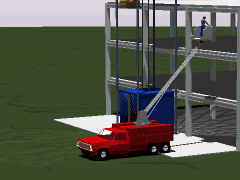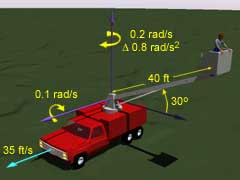Ch 9. 3-D Motion of a Rigid Body Multimedia Engineering Dynamics Translating Coordinates Rotating Coordinates Inertia & Ang. Momentum Equationsof Motion
 Chapter - Particle - 1. General Motion 2. Force & Accel. 3. Energy 4. Momentum - Rigid Body - 5. General Motion 6. Force & Accel. 7. Energy 8. Momentum 9. 3-D Motion 10. Vibrations Appendix Basic Math Units Basic Equations Sections Search eBooks Dynamics Fluids Math Mechanics Statics Thermodynamics Author(s): Kurt Gramoll ©Kurt GramollDYNAMICS - CASE STUDYProblem Graphic Introduction As the utility truck pulls away, the worker at the top of the arm rotates the arm down and 180° toward the front of the truck. If the acceleration is too great, he may fall off the truck. What is known: All values are given at the instant the arm is perpendicular to the path of the truck. The truck is traveling in a straight line at a constant rate of 35 ft/s. The arm is 40 ft long, and is 30° up from horizontal. The arm is rotating about a vertical axis toward the front of the truck at a rate of 0.2 rad/s, increasing at 0.8 rad/s2. The arm is rotating about a horizontal axis parallel to the path of the truck at a constant rate of 0.1 rad/s. QuestionProblem Diagram When the arm is perpendicular to the path of the truck, what are the velocity and acceleration of the worker with respect to the ground? Approach Consider a translating coordinate system located at the base of the arm. Use the equations of general motion in three dimensions to determine the motion of the end of the arm with respect to a fixed (non-translating) coordinate system. Only consider the instant in time when the arm is perpendicular to the path of the truck.

Practice Homework and Test problems now available in the 'Eng Dynamics' mobile app
Includes over 400 problems with complete detailed solutions.
Available now at the Google Play Store and Apple App Store.